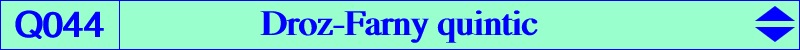X(2), X(4), X(671), X(1113), X(1114) Ha, Hb, Hc : feet of the altitudes Ga, Gb, Gc : vertices of the antimedial triangle A4, B4, C4 : vertices of the fourth Brocard triangle (projections of H on the medians) X4-OAP points, see also Table 53. too complicated to be written here. Click on the link to download a text file.The Droz-Farny quintic Q044 is the locus of P such that the trilinear polar of P and the Droz-Farny axis of P are parallel. See CL039. Q044 is an antigonal circular quintic with three real asymptotes parallel at G to those of the Thomson cubic K002. The two other imaginary asymptotes intersect at X(381), the midpoint of GH. Q044 has four nodes A, B, C, H. The nodal tangents at A are the symmedian AK and the line GbGc. The nodal tangents at H are parallel to the asymptotes of the Jerabek hyperbola. The tangents at Ha, Hb, Hc concur at G. The tangents at Ga, Gb, Gc concur at X(315). The tangent at G is the line X(2)X(99)X(671). Q044 is the locus of point Q such that Q, its antigonal and G are collinear.